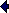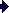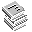Aerostatic thrust bearing with grouped inherent orifices

Diameter bearing 2R0   10-3 m
Supply pressure pS   106 Pa
Ambient pressure pA   106 Pa
Pressure factor βLoad capacity F*

Pressure ratio pR/pS
Load capacity F   N

 F*: Is usually taken from design charts or calculated numerically. The F*-value in this calculator is calculated numerically with R1/R0=0.5 and β=0.6.www.tribology-abc.com